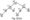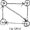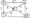# Analysis and Design of Algorithms Dec 2010

Note: Answer any FIVE full questions,

selecting at least TWO questions from each part

PART-A

1 a. What is an algorithm? With a neat diagram, explain the algorithm design and analysis process.

b. Define the asymptotic notations for worst case, best case and average case time

complexities. Give example.

2 a.What suitable example Explain the significance of the order of growth analyzing the algorithm efficiency.

b.  Prove that:                 i) Jn(n-l) e 9(n2) ii) n! e Q (2n).

c.   Distinguish between the two common ways to represent a graph — given the representation of undirected graph. Explain how the following can be ascertained by the representation

i) The graph is completed ii) The graph has a loop iii) The graph has an isolated vertex Answer for each of the representation separately.

3  a. What is a “Bruteforce” method? Under what condition does the method become desirable?

b.   State the merge sort algorithm and analyze its complexity.

c.   Outline an exhaustive search algorithm to solve the traveling salesman problem.

4 a. Briefly explain the strassen’s matrix multiplication. How it uses divide and conquer method? Obtain its time complexity.

b. Write an algorithm to topologically sort a diagrph using DFS. Prove the correctness of the algorithm, with examples.

c.   With the suitable example, explain the Johnson trotter algorithm, to generate the permutation of given objects.

PART-B

5 a. What is an AVL tree? Explain the four types of rotations used to construct the AVL tree. Insert 1, 25, 28, and 12 in the following tree.b.  When does collision occur in hashing? Arrange the following keys in the hash table of size 10, using open hashing technique. A, GOOD, STUDENT, WORKS, HARD. (04 Marks)

c.   Construct a Huffman code for the following data :

 Char A B c D E Probability 0.4 0.1 0.25 0.2 0.15

i)   Encode the text ABACABAD using generated code

ii) Decode the text whose encoding is 10001011100101.

6 a.With the help of pseudocode, explain Marshall’s algorithm to find the transitive closure of a directed graph. Apply it to the graph shown below :b. Find the minimum spanning tree, using Prim’s method for the graph shown below:Fig. Q6(b)

7 a. Find the single source shortest paths. Apply the Dijkstra’s algorithm to Fig. Q7(a), to get the shortest path form the vertex to all the other vertices,b. Write a bottom-up dynamic algorithm for the knapsack problem. Apply it on the following instance of the knapsack problem.

 Item Weight Value 1 2 3 2 3 4 3 4 5 4 5 6 fable Q7(b)

8 a. Define P, NP and NP complete problem.

b. Explain how the TSP problem can be solved using branch and bound methods.

c.   Explain the back – traching algorithm. Apply the same to solve the following instance of the subset sum problem

s – {5, 10, 12, 13, 15, 18} and d = 30.

### 1 thought on “VTU Previous question Papers BE CS 4th Semester Analysis and Design of Algorithms Dec 2010”

1.This is really interesting, You are an excessively professional blogger. I’ve joined your rss feed and sit up for searching for more of your fantastic post. Additionally, I have shared your web site in my social networks!